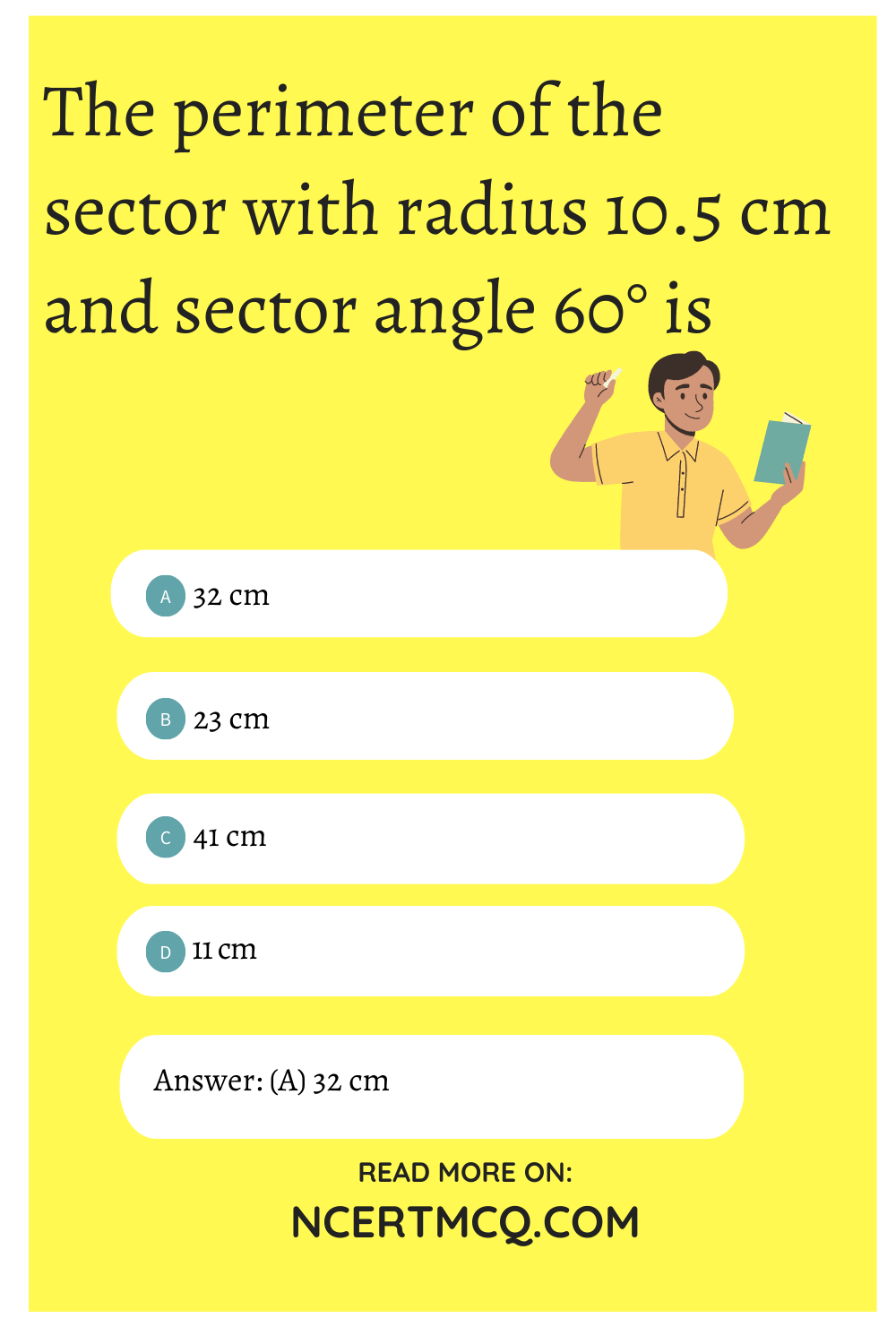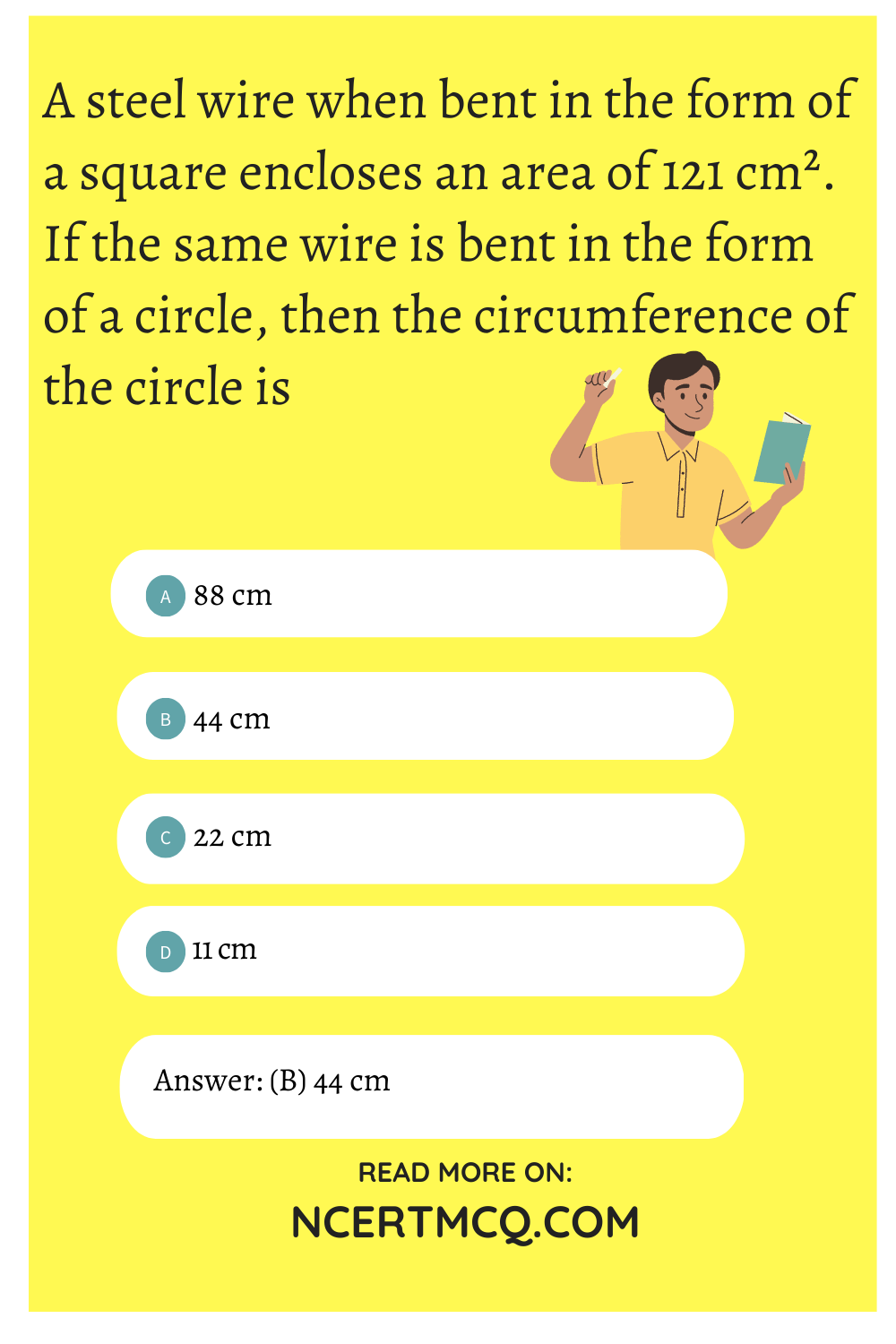Check the below NCERT MCQ Questions for Class 10 Maths Chapter 12 Areas Related to Circles with Answers Pdf free download. MCQ Questions for Class 10 Maths with Answers were prepared based on the latest exam pattern. We have provided Areas Related to Circles Class 10 Maths MCQs Questions with Answers to help students understand the concept very well. https://ncertmcq.com/mcq-questions-for-class-10-maths-with-answers/

Students can also refer to NCERT Solutions for Class 10 Maths Chapter 12 Areas Related to Circles for better exam preparation and score more marks.

## Areas Related to Circles Class 10 MCQs Questions with Answers

Area Related To Circle Class 10 MCQ Question 1.
Perimeter of a sector of a circle whose central angle is 90° and radius 7 cm is
(a) 35 cm
(b) 25 cm
(c) 77 cm
(d) 7 cm

Areas Related To Circles Class 10 MCQ Question 2.
The area of a circle that can be inscribed in a square of side 10 cm is
(a) 40π cm²
(b) 30π cm²
(c) 100π cm²
(d) 25π cm²

Class 10 Maths Chapter 12 MCQ Question 3.
The perimeter of a square circumscribing a circle of radius a units is
(a) 2 units
(b) 4α units
(c) 8α units
(d) 16α units

Areas Related To Circles Class 10 MCQ With Answers Question 4.
The perimeter of the sector with radius 10.5 cm and sector angle 60° is
(a) 32 cm
(b) 23 cm
(c) 41 cm
(d) 11 cmMCQ Questions For Class 10 Maths Areas Related To Circles Question 5.
In a circle of diameter 42 cm, if an arc subtends an angle of 60° at the centre, where π = $$\frac{22}{7}$$ then length of arc is
(a) 11 cm
(b) $$\frac{22}{7}$$ cm
(c) 22 cm
(d) 44 cm

Ch 12 Maths Class 10 MCQ Question 6.
The perimeter of a sector of radius 5.2 cm is 16.4 cm, the area of the sector is
(a) 31.2 cm²
(b) 15 cm²
(c) 15.6 cm²
(d) 16.6 cm²

Class 10 Area Related To Circle MCQ Question 7.
If the perimeter of a semicircular protractor is 72 cm where π = $$\frac{22}{7}$$, then the diameter of protractor is
(a) 14 cm
(b) 33 cm
(c) 28 cm
(d) 42 cm

MCQ Area Related To Circle Class 10 Question 8.
If the radius of a circle is doubled, its area becomes
(a) 2 times
(b) 4 times
(c) 8 times
(d) 16 times

Class 10 Areas Related To Circles MCQ Question 9.
If the sum of the circumferences of two circles with radii R1 and R2 is equal to circumference of a circle of radius R, then
(a) R1 + R2 = R
(b) R1 + R2 > R
(c) R1 + R2 < R
(d) Can’t say;

Answer: (a) R1 + R2 = R

MCQ On Areas Related To Circles Class 10 Question 10.
The perimeter of a circular and square fields are equal. If the area of the square field is 484 m² then the diameter of the circular field is
(a) 14 m
(b) 21 m
(c) 28 m
(d) 7 m

Question 11.
The radius of sphere is r cm. It is divided into . two equal parts. The whole surface area of two parts will be
(a) 8πr² cm²
(b) 6πr² cm²
(c) 4πr² cm²
(d) 3πr² cm²

Question 12.
If the diameter of a semicircular protractor is 14 cm, then its perimeter is .
(a) 27 cm
(b) 36 cm
(c) 18 cm
(d) 9 cm

Question 13.
A race track is in the form of a circular ring whose outer and inner circumferences are 396 m and 352 m respectively. The width of the track is
(a) 63 m
(b) 56 m
(c) 7 m
(d) 3.5 m

Question 14.
The area of the largest square that can be inscribed in a circle of radius 12 cm is
(a) 24 cm²
(b) 249 cm²
(c) 288 cm²
(d) 196√2 cm²

Question 15.
The area of the largest triangle that can be inscribed in a semicircle of radius r is
(a) r²
(b) 2r²
(c) r³
(d) 2r³

Question 16.
The area (in cm²) of the circle that can be inscribed in a square of side 8 cm is
(a) 64 π
(b) 16 π
(c) 8 π
(d) 32 π

Question 17.
If the perimeter of a circle is equal to that of a square, then the ratio of their areas is
(a) 22 : 7
(b) 14 : 11
(c) 7 : 22
(d) 11 : 14

Question 18.
The circumference of two concentric circles forming a ring are 88 cm and 66 cm. Taking π = $$\frac{22}{7}$$, the width of the ring is
(a) 14 cm
(b) 7 cm
(c) $$\frac{7}{2}$$ cm
(d) 21 cm

Answer: (c) $$\frac{7}{2}$$ cm

Question 19.
A steel wire when bent in the form of a square encloses an area of 121 cm². If the same wire is bent in the form of a circle, then the circumference of the circle is
(a) 88 cm
(b) 44 cm
(c) 22 cm
(d) 11 cmQuestion 20.
The diameter of a circle whose area is equal to sum of the areas of the two circles of radii 40 cm and 9 cm is
(a) 41 cm
(b) 49 cm
(c) 82 cm
(d) 62 cm